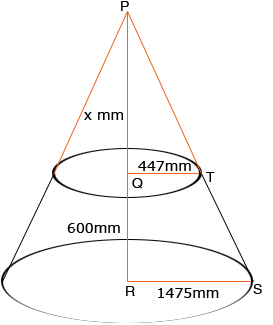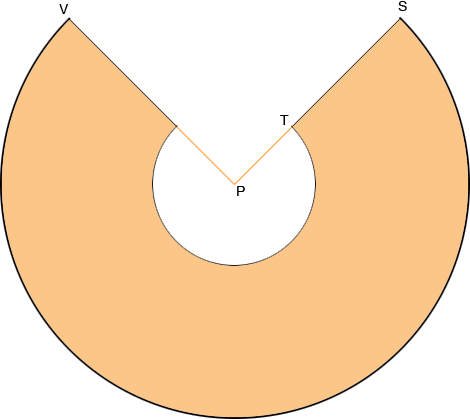Subject: cone Name: suresh Who are you: Other (All) dear sir i want to know the required size of plate for cone rolling,sizes are 2950mm is bottom dia,894 is top dia and 600 is height.I have already read u r answers but i little bit confused ,harely and sue have given useful answers but when i worked both method the required plate size is different. so i like to know which method is easy and correct.and also i like know whether it can be rolled without segment my rolling machine width is 1500.I am waiting for u r reply please. THANK YOU Suresh, I drew a picture of your truncated cone and extended it to a vertex P.In the diagram the distance PQ is x mm. Using similar triangles  (600 + x)/1475 = x/447   Solving for x I found that x = 260.89 mm. Using Pythagoras theorem with the triangles PQT and PRS I found |PT| = 517.56 mm and |PS| = 1707.85 mm. Thus the plate can be contructed from a disk of radius |PS| = 1707.85 mm by removing a cisk of radius |PT| = 517.56 mm.Using the same method as in the answer to a previous problem I found the angle VPS to be 49.09 degrees. Penny